Common Lisp the Language, 2nd EditionNext: Type Conversions and Up: Irrational and Transcendental Previous: Trigonometric and Related

## 12.5.3. Branch Cuts, Principal Values, and Boundary Conditions in the Complex Plane

Many of the irrational and transcendental functions are multiply defined in the complex domain; for example, there are in general an infinite number of complex values for the logarithm function. In each such case, a principal value must be chosen for the function to return. In general, such values cannot be chosen so as to make the range continuous; lines in the domain called branch cuts must be defined, which in turn define the discontinuities in the range.

Common Lisp defines the branch cuts, principal values, and boundary conditions for the complex functions following a proposal for complex functions in APL . The contents of this section are borrowed largely from that proposal.

Compatibility note: The branch cuts defined here differ in a few very minor respects from those advanced by W. Kahan, who considers not only the ``usual'' definitions but also the special modifications necessary for IEEE proposed floating-point arithmetic, which has infinities and minus zero as explicit computational objects. For example, he proposes that SQRT(-4+0i)=2i, but SQRT(-4-0i)=-2i.

It may be that the differences between the APL proposal and Kahan's proposal will be ironed out. If so, Common Lisp may be changed as necessary to be compatible with these other groups. Any changes from the specification below are likely to be quite minor, probably concerning primarily questions of which side of a branch cut is continuous with the cut itself.Indeed, X3J13 voted in January 1989 (COMPLEX-ATAN-BRANCH-CUT)   to alter the direction of continuity for the branch cuts of atan, and also (IEEE-ATAN-BRANCH-CUT)   to address the treatment of branch cuts in implementations that have a distinct floating-point minus zero.

The treatment of minus zero centers in two-argument atan. If there is no minus zero, then the branch cut runs just below the negative real axis as before, and the range of two-argument atan is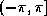. If there is a minus zero, however, then the branch cut runs precisely on the negative real axis, skittering between pairs of numbers of the form -x+/-0i, and the range of two-argument atan is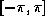.

The treatment of minus zero by all other irrational and transcendental functions is then specified by defining those functions in terms of two-argument atan. First, phase is defined in terms of two-argument atan, and complex abs in terms of real sqrt; then complex log is defined in terms of phase, abs, and real log; then complex sqrt in terms of complex log; and finally all others are defined in terms of these.

Kahan  treats these matters in some detail and also suggests specific algorithms for implementing irrational and transcendental functions in IEEE standard floating-point arithmetic .

Remarks in the first edition about the direction of the continuity of branch cuts continue to hold in the absence of minus zero and may be ignored if minus zero is supported; since all branch cuts happen to run along the principal axes, they run between plus zero and minus zero, and so each sort of zero is associated with the obvious quadrant.sqrt
The branch cut for square root lies along the negative real axis, continuous with quadrant II. The range consists of the right half-plane, including the non-negative imaginary axis and excluding the negative imaginary axis.X3J13 voted in January 1989 (IEEE-ATAN-BRANCH-CUT)   to specify certain floating-point behavior when minus zero is supported. As a part of that vote it approved a mathematical definition of complex square root:
```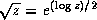```
This defines the branch cuts precisely, whether minus zero is supported or not.phase
The branch cut for the phase function lies along the negative real axis, continuous with quadrant II. The range consists of that portion of the real axis between -pi (exclusive) and pi (inclusive).X3J13 voted in January 1989 (IEEE-ATAN-BRANCH-CUT)   to specify certain floating-point behavior when minus zero is supported. As a part of that vote it approved a mathematical definition of phase:
```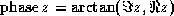```
where Fz is the imaginary part of z and Rz the real part of z. This defines the branch cuts precisely, whether minus zero is supported or not.log
The branch cut for the logarithm function of one argument (natural logarithm) lies along the negative real axis, continuous with quadrant II. The domain excludes the origin. For a complex number z, z is defined to be
``` log z = (log|z|) + i(phase z)
```
Therefore the range of the one-argument logarithm function is that strip of the complex plane containing numbers with imaginary parts between -pi (exclusive) and pi (inclusive).The X3J13 vote on minus zero (IEEE-ATAN-BRANCH-CUT)   would alter that exclusive bound of -pi to be inclusive if minus zero is supported.The two-argument logarithm function is defined as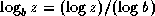. This defines the principal values precisely. The range of the two-argument logarithm function is the entire complex plane. It is an error if z is zero. If z is non-zero and b is zero, the logarithm is taken to be zero.

exp
The simple exponential function has no branch cut.

expt
The two-argument exponential function is defined as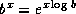. This defines the principal values precisely. The range of the two-argument exponential function is the entire complex plane. Regarded as a function of x, with b fixed, there is no branch cut. Regarded as a function of b, with x fixed, there is in general a branch cut along the negative real axis, continuous with quadrant II. The domain excludes the origin. By definition,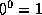. If b=0 and the real part of x is strictly positive, then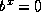. For all other values of x,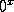is an error.

asin
The following definition for arc sine determines the range and branch cuts:
```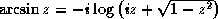```
This is equivalent to the formula
```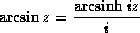```
recommended by Kahan .

The branch cut for the arc sine function is in two pieces: one along the negative real axis to the left of -1 (inclusive), continuous with quadrant II, and one along the positive real axis to the right of 1 (inclusive), continuous with quadrant IV. The range is that strip of the complex plane containing numbers whose real part is between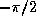and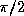. A number with real part equal tois in the range if and only if its imaginary part is non-negative; a number with real part equal tois in the range if and only if its imaginary part is non-positive.

acos
The following definition for arc cosine determines the range and branch cuts:
```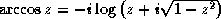```
or, which is equivalent,
```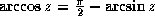```
The branch cut for the arc cosine function is in two pieces: one along the negative real axis to the left of -1 (inclusive), continuous with quadrant II, and one along the positive real axis to the right of 1 (inclusive), continuous with quadrant IV. This is the same branch cut as for arc sine. The range is that strip of the complex plane containing numbers whose real part is between zero and pi. A number with real part equal to zero is in the range if and only if its imaginary part is non-negative; a number with real part equal to pi is in the range if and only if its imaginary part is non-positive.

atan
The following definition for (one-argument) arc tangent determines the range and branch cuts:
```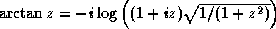```Beware of simplifying this formula; ``obvious'' simplifications are likely to alter the branch cuts or the values on the branch cuts incorrectly.

The branch cut for the arc tangent function is in two pieces: one along the positive imaginary axis above i (exclusive), continuous with quadrant II, and one along the negative imaginary axis below -i (exclusive), continuous with quadrant IV. The points i and -i are excluded from the domain. The range is that strip of the complex plane containing numbers whose real part is betweenand. A number with real part equal tois in the range if and only if its imaginary part is strictly positive; a number with real part equal tois in the range if and only if its imaginary part is strictly negative. Thus the range of the arc tangent function is identical to that of the arc sine function with the pointsandexcluded.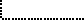X3J13 voted in January 1989 (COMPLEX-ATAN-BRANCH-CUT)   to replace the formula shown above with the formula
```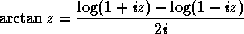```
This is equivalent to the formula
```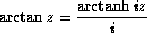```
recommended by Kahan . It causes the upper branch cut to be continuous with quadrant I rather than quadrant II, and the lower branch cut to be continuous with quadrant III rather than quadrant IV; otherwise it agrees with the formula of the first edition. Therefore this change alters the result returned by atan only for arguments on the positive imaginary axis that are of magnitude greater than 1. The full description for this new formula is as follows.

The branch cut for the arc tangent function is in two pieces: one along the positive imaginary axis above i (exclusive), continuous with quadrant I, and one along the negative imaginary axis below -i (exclusive), continuous with quadrant III. The points i and -i are excluded from the domain. The range is that strip of the complex plane containing numbers whose real part is betweenand. A number with real part equal tois in the range if and only if its imaginary part is strictly negative; a number with real part equal tois in the range if and only if its imaginary part is strictly positive. Thus the range of the arc tangent function is not identical to that of the arc sine function.asinh
The following definition for the inverse hyperbolic sine determines the range and branch cuts:
```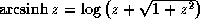```
The branch cut for the inverse hyperbolic sine function is in two pieces: one along the positive imaginary axis above i (inclusive), continuous with quadrant I, and one along the negative imaginary axis below -i (inclusive), continuous with quadrant III. The range is that strip of the complex plane containing numbers whose imaginary part is betweenand. A number with imaginary part equal tois in the range if and only if its real part is non-positive; a number with imaginary part equal tois in the range if and only if its real part is non-negative.

acosh
The following definition for the inverse hyperbolic cosine determines the range and branch cuts:
```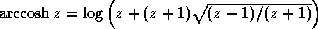```Kahan  suggests the formula
```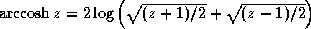```
pointing out that it yields the same principal value but eliminates a gratuitous removable singularity at z=-1. A proposal was submitted to X3J13 in September 1989 to replace the formula acosh with that recommended by Kahan. There is a good possibility that it will be adopted.The branch cut for the inverse hyperbolic cosine function lies along the real axis to the left of 1 (inclusive), extending indefinitely along the negative real axis, continuous with quadrant II and (between 0 and 1) with quadrant I. The range is that half-strip of the complex plane containing numbers whose real part is non-negative and whose imaginary part is between -pi (exclusive) and pi (inclusive). A number with real part zero is in the range if its imaginary part is between zero (inclusive) and pi (inclusive).

atanh
The following definition for the inverse hyperbolic tangent determines the range and branch cuts:```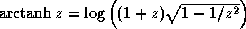WRONG!
```WARNING! The formula shown above for hyperbolic arc tangent is incorrect. It is not a matter of incorrect branch cuts; it simply does not compute anything like a hyperbolic arc tangent. This unfortunate error in the first edition was the result of mistranscribing a (correct) APL formula from Penfield's paper . The formula should have been transcribed as
```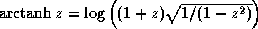```Beware of simplifying this formula; ``obvious'' simplifications are likely to alter the branch cuts or the values on the branch cuts incorrectly.

The branch cut for the inverse hyperbolic tangent function is in two pieces: one along the negative real axis to the left of -1 (inclusive), continuous with quadrant III, and one along the positive real axis to the right of 1 (inclusive), continuous with quadrant I. The points -1 and 1 are excluded from the domain. The range is that strip of the complex plane containing numbers whose imaginary part is betweenand. A number with imaginary part equal tois in the range if and only if its real part is strictly negative; a number with imaginary part equal tois in the range if and only if its real part is strictly positive. Thus the range of the inverse hyperbolic tangent function is identical to that of the inverse hyperbolic sine function with the points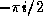and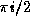excluded.A proposal was submitted to X3J13 in September 1989 to replace the formula atanh with that recommended by Kahan :
```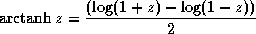```
There is a good possibility that it will be adopted. If it is, the complete description of the branch cuts of atanh will then be as follows.

The branch cut for the inverse hyperbolic tangent function is in two pieces: one along the negative real axis to the left of -1 (inclusive), continuous with quadrant II, and one along the positive real axis to the right of 1 (inclusive), continuous with quadrant IV. The points -1 and 1 are excluded from the domain. The range is that strip of the complex plane containing numbers whose imaginary part is betweenand. A number with imaginary part equal tois in the range if and only if its real part is strictly positive; a number with imaginary part equal tois in the range if and only if its real part is strictly negative. Thus the range of the inverse hyperbolic tangent function is not the same as that of the inverse hyperbolic sine function.With these definitions, the following useful identities are obeyed throughout the applicable portion of the complex domain, even on the branch cuts:

```sin iz = i sinh z  sinh iz = i sin z        arctan iz = i arctanh z
cos iz = cosh z    cosh iz = cos z          arcsinh iz = i arcsin z
tan iz = i tanh z  arcsin iz = i arcsinh z  arctanh iz = i arctan z
```I thought it would be useful to provide some graphs illustrating the behavior of the irrational and transcendental functions in the complex plane. It also provides an opportunity to show off the Common Lisp code that was used to generate them.

Imagine the complex plane to be decorated as follows. The real and imaginary axes are painted with thick lines. Parallels from the axes on both sides at distances of 1, 2, and 3 are painted with thin lines; these parallels are doubly infinite lines, as are the axes. Four annuli (rings) are painted in gradated shades of gray. Ring 1, the inner ring, consists of points whose radial distances from the origin lie in the range [1/4, 1/2]; ring 2 is in the radial range [3/4, 1]; ring 3, in the range [pi/2, 2]; and ring 4, in the range [3, pi]. Ring j is divided into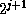equal sectors, with each sector painted a different shade of gray, darkening as one proceeds counterclockwise from the positive real axis.

We can illustrate the behavior of a numerical function f by considering how it maps the complex plane to itself. More specifically, consider each point z of the decorated plane. We decorate a new plane by coloring the point f(z) with the same color that point z had in the original decorated plane. In other words, the newly decorated plane illustrates how the f maps the axes, other horizontal and vertical lines, and annuli.

In each figure we will show only a fragment of the complex plane, with the real axis horizontal in the usual manner (-pi to the left, +pi to the right) and the imaginary axis vertical (-i below, +pii above). Each fragment shows a region containing points whose real and imaginary parts are in the range [-4.1, 4.1]. The axes of the new plane are shown as very thin lines, with large tick marks at integer coordinates and somewhat smaller tick marks at multiples of.

Figure 12-1 shows the result of plotting the identity function (quite literally); the graph exhibits the decoration of the original plane.

Figures 12-2 through 12-20 show the graphs for the functions sqrt, exp, log, sin, asin, cos, acos, tan, atan, sinh, asinh, cosh, acosh, tanh, and atanh, and as a bonus, the graphs for the functions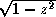,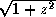,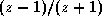, and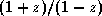. All of these are related to the trigonometric functions in various ways. For example, if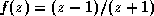, then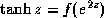, and if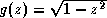, then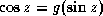. It is instructive to examine the graph forand try to visualize how it transforms the graph for sin into the graph for cos.

Each figure is accompanied by a commentary on what maps to what and other interesting features. None of this material is terribly new; much of it may be found in any good textbook on complex analysis. I believe that the particular form in which the graphs are presented is novel, as well as the fact that the graphs have been generated as PostScript  code by Common Lisp code. This PostScript code was then fed directly to the typesetting equipment that set the pages for this book. Samples of this PostScript code follow the figures themselves, after which the code for the entire program is presented.

In the commentaries that accompany the figures I sometimes speak of mapping the points +/- infinity or +/- infinity i. When I say that function f maps +infinity to a certain point z, I mean that

```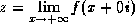```
Similarly, when I say that f maps -i to z, I mean that
```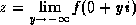```
In other words, I am considering a limit as one travels out along one of the main axes. I also speak in a similar manner of mapping to one of these infinities.

Here is a sample of the PostScript code that generated figure 12-1, showing the initial scaling, translation, and clipping parameters; the code for one sector of the innermost annulus; and the code for the negative imaginary axis. Comment lines indicate how path or boundary segments were generated separately and then spliced (in order to allow for the places that a singularity might lurk, in which case the generating code can ``inch up'' to the problematical argument value).

The size of the entire PostScript file for the identity function was about 68 kilobytes (2757 lines, including comments). The smallest files were the plots for atan and atanh, about 65 kilobytes apiece; the largest were the plots for sin, cos, sinh, and cosh, about 138 kilobytes apiece.

% PostScript file for plot of function IDENTITY % Plot is to fit in a region 4.666666666666667 inches square % showing axes extending 4.1 units from the origin. 40.97560975609756 40.97560975609756 scale 4.1 4.1 translate newpath -4.1 -4.1 moveto 4.1 -4.1 lineto 4.1 4.1 lineto -4.1 4.1 lineto closepath clip % Moby grid for function IDENTITY % Annulus 0.25 0.5 4 0.97 0.45 % Sector from 4.7124 to 6.2832 (quadrant 3) newpath 0.0 -0.25 moveto 0.0 -0.375 lineto %middle radial 0.0 -0.375 lineto 0.0 -0.5 lineto %end radial 0.0 -0.5 lineto 0.092 -0.4915 lineto 0.1843 -0.4648 lineto 0.273 -0.4189 lineto 0.3536 -0.3536 lineto %middle circumferential 0.3536 -0.3536 lineto 0.413 -0.2818 lineto 0.4594 -0.1974 lineto 0.4894 -0.1024 lineto 0.5 0.0 lineto %end circumferential 0.5 0.0 lineto 0.375 0.0 lineto %middle radial 0.375 0.0 lineto 0.25 0.0 lineto %end radial 0.25 0.0 lineto 0.2297 -0.0987 lineto 0.1768 -0.1768 lineto %middle circumferential 0.1768 -0.1768 lineto 0.0922 -0.2324 lineto 0.0 -0.25 lineto %end circumferential closepath currentgray 0.45 setgray fill setgray

[2598 lines omitted]

% Vertical line from (0.0, -0.5) to (0.0, 0.0) newpath 0.0 -0.5 moveto 0.0 0.0 lineto 0.05 setlinewidth 1 setlinecap stroke % Vertical line from (0.0, -0.5) to (0.0, -1.0) newpath 0.0 -0.5 moveto 0.0 -1.0 lineto 0.05 setlinewidth 1 setlinecap stroke % Vertical line from (0.0, -2.0) to (0.0, -1.0) newpath 0.0 -2.0 moveto 0.0 -1.0 lineto 0.05 setlinewidth 1 setlinecap stroke % Vertical line from (0.0, -2.0) to (0.0, -1.1579208923731617E77) newpath 0.0 -2.0 moveto 0.0 -6.3553 lineto 0.0 -6.378103166302659 lineto 0.0 -6.378103166302659 lineto 0.0 -6.378103166302659 lineto 0.05 setlinewidth 1 setlinecap stroke

[84 lines omitted]

% End of PostScript file for plot of function IDENTITY

Here is the program that generated the PostScript code for the graphs shown in figures 12-1 through 12-20. It contains a mixture of fairly general mechanisms and ad hoc kludges for plotting functions of a single complex argument while gracefully handling extremely large and small values, branch cuts, singularities, and periodic behavior. The aim was to provide a simple user interface that would not require the caller to provide special advice for each function to be plotted. The file for figure 12-1, for example, was generated by the call (picture 'identity), which resulted in the writing of a file named identity-plot.ps.

The program assumes that any periodic behavior will have a period that is a multiple of 2pi; that branch cuts will fall along the real or imaginary axis; and that singularities or very large or small values will occur only at the origin, at 1 or +/-i, or on the boundaries of the annuli (particularly those with radiusor pi). The central function is parametric-path, which accepts four arguments: two real numbers that are the endpoints of an interval of real numbers, a function that maps this interval into a path in the complex plane, and the function to be plotted; the task of parametric-path is to generate PostScript code (a series of lineto operations) that will plot an approximation to the image of the parametric path as transformed by the function to be plotted. Each of the functions hline, vline, -hline, -vline, radial, and circumferential takes appropriate parameters and returns a function suitable for use as the third argument to parametric-path. There is some code that defends against errors (by using ignore-errors) and against certain peculiarities of IEEE floating-point arithmetic (the code that checks for not-a-number (NaN) results).

The program is offered here without further comment or apology.